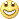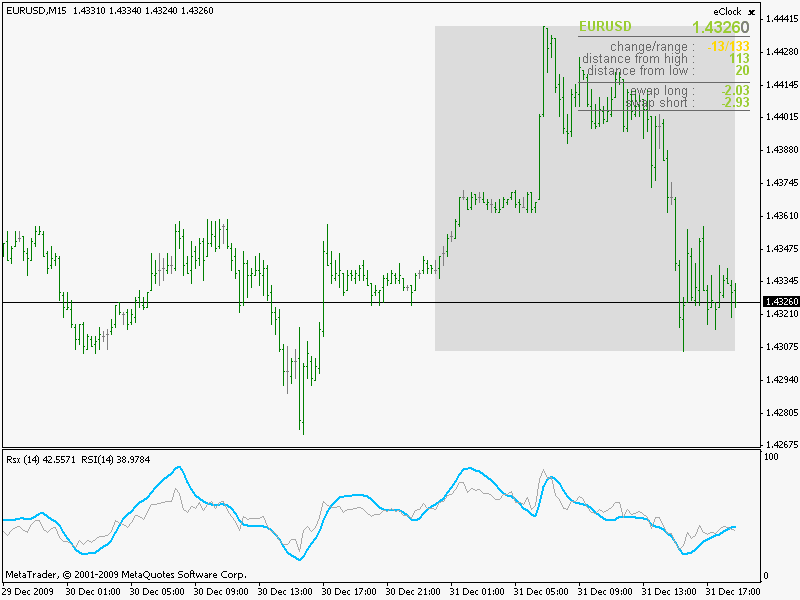# Dynamic zone indicators ...222262

It seems that David Stendahl can not help him selfWhere his name appears "attached" to some indicator or TA way, it sure is worth trying it out and studying it.

The basis for these were originally published in the Stocks & Commodities July 1996 issue (attached the original article here). Do not be confused by the name as I was. Indicators called "dynamic zone" (dynamic zone RSI for example - one version of it can be found here : https://www.mql5.com/en/forum/173787) have almost nothing common at all with these indicators. The "dynamic zone" indicators floating on the net are a simple bolinger bands applied to some indicator while, according to Leo Zamansky and David Stendahl we have :

To better understand dynamic zones, let�s first describe them mathematically and then explain their use. The dynamic zones definition:
Find V such that:

For dynamic zone buy: P{X <= V}=P1

For dynamic zone sell: P{X >= V}=P2
where P1 and P2 are the probabilities set by the trader, X is the value of the indicator for the selected period and V represents the value of the dynamic zone.

The probability input P1 and P2 can be adjusted by the trader to encompass as much or as little data as the trader would like. The smaller the probability, the fewer data values above and below the dynamic zones. This translates into a wider range between the buy and sell zones. If a 10% probability is used for P1 and P2, only those data values that make up the top 10% and bottom 10% for an indicator are used in the construction of the zones. Of the values, 80% will fall between the two extreme levels. Because dynamic zone levels are penetrated so infrequently, when this happens, traders know that the market has truly moved into overbought or oversold territory.

CALCULATING THE DYNAMIC ZONES

The algorithm for the dynamic zones is a series of steps. First, decide the value of the lookback period t. Next, decide the value of the probability Pbuy for buy zone and value of the probability Psell for the sell zone.

For i=1, to the last lookback period, build the distribution f(x) of the price during the lookback period i. Then find the value Vi1 such that the probability of the price less than or equal to Vi1 during the lookback period i is equal to Pbuy. Find the value Vi2 such that the probability of the price greater or equal to Vi2 during the lookback period i is equal to Psell. The sequence of Vi1 for all periods gives the buy zone. The sequence of Vi2 for all periods gives the sell zone.

In the algorithm description, we have: �Build the distribution f(x) of the price during the lookback period i.� The distribution here is empirical � namely, how many times a given value of x appeared during the lookback period. The problem is to find such x that the probability of a price being

greater or equal to x will be equal to a probability selected by the user. Probability is the area under the distribution curve. The task is to find such value of x that the area under the distribution curve to the right of x will be equal to the probability selected by the user. That x is the dynamic zone.

So, nothing to do with Bollinger bandsDynamic zone calculation can be applied to a wide range of indicators and this thread is going to be a thread where I think those should be kept (simply in order to make them available in one place)

____________________

PS: attached here the dynamicZone.dll (in the zip file) so that it is not necessary to attach it to every post that uses it. The last version of it will always be posted here. If it is going to be changed, backwards comp ability is going to be preserved

PPS: the latest version of dll is in the "dynamicZone dll May 2014.zip" file. Download it and overwrite the old one. All the old indicator will work with the new dll too

dynamiczone_dll_may_2014.zip

Files:9576

Dynamically dynamic Mladen !!Great work!222262

Dynamic zone synthetic RSI

Dynamic zone synthetic RSI

___________________________

Parameters :
RsiPrice- price to apply to

EmaLength1- length for long EMA pre-RSI calculation smoothing

RsiLength1- length for long RSI calculation

EmaLength2- length for mid length EMA pre-RSI calculation smoothing

RsiLength2- length for mid length RSI calculation

EmaLength3- length for short EMA pre-RSI calculation smoothing

RsiLength3- length for short RSI calculation

DzLookBackBars- number of bars to look back for dynamic zone

DzStartBuyProbability- probability for buy zone

DzStartSellProbability- probability for sell zone

___________________________

Posting here two versions of the indicator. The "classical" ("all metatrader code" version) and the one that uses dll for finding out dynamic zones. The dll version solves the problem that long lookback dynamic zones and a multiple dynamic zones can have : "processor hungry". Dll version does the job much faster and thus it gives more freedom as of how many zones to use. There is no value difference between the two (so they give exactly the same values, the difference is in the speed of execution)

PS: the dynamicZone.dll (posted at post #1 of this thread) should go to the libraries folder

Files:222262

Dynamic zone synthetic smoothed RSI

Dynamic zone synthetic smoothed RSI

___________________________

Parameters :
RsiPrice- price to apply to

EmaLength1- length for long EMA pre-RSI calculation smoothing

RsiLength1- length for long RSI calculation

EmaLength2- length for mid length EMA pre-RSI calculation smoothing

RsiLength2- length for mid length RSI calculation

EmaLength3- length for short EMA pre-RSI calculation smoothing

RsiLength3- length for short RSI calculation

DzLookBackBars- number of bars to look back for dynamic zone

DzStartBuyProbability- probability for buy zone

DzStartSellProbability- probability for sell zone

SmoothedRsi- calculate smoothed rsi or not

___________________________

PS: the dynamicZone.dll (posted at post #1 of this thread) should go to the libraries folder

Files:222262

Dynamic zone RSX

Dynamic zone RSX

According to Mark Jurik, RSX is a "no lagging, smoothed RSI" (which is, more or lees, truth. See the picture for comparison of regular RSI (gray) and RSX (blue)) so it is logical that we should have this one here too.He also had an idea to use cfb in order to make it cross the zero line faster. This one, instead of speeding up the rsx movement toward zero line, moves the zero line (the beauty of the dynamic zone concept), so the effect is almost the same___________________________

Parameters :
RsxLength- length for RSX calculation

RsxPrice- price for RSX calculationDzLookBackBars- number of bars to look back for dynamic zone

DzStartBuyProbability- probability for buy zone

DzStartSellProbability- probability for sell zone

___________________________

PS: the dynamicZone.dll (posted at post #1 of this thread) should go to the libraries folder

Files:
dz_rsx.gif  16 kb85

Hello Mladen,

the dynamic zone is perfect but it is possible to have dynamic zone stochastic?

I use a lot with this indicator levels 20-80 but have dynamic zone might be better!

Regards222262

Dynamic zone stochastic

Here you goDid not make it because its similarities with WPR, but it is much better to have it as a standalone indicator

___________________________

Parameters :
StoKPeriod- stochastic K period

StoDPeriod- stochastic D period

StoSlowingPeriod- stochastic slowing period

StoMaMethod- moving average method to use

StoPrice- prices to use in calculation
0 - High/Low prices

1 - Close/Close prices

DzLookBackBars- number of bars to look back for dynamic zone

DzStartBuyProbability- probability for buy zone

DzStartSellProbability- probability for sell zone

___________________________

PS: the dynamicZone.dll (posted at post #1 of this thread) should go to the libraries folder

regards

mladen

gafet:
Hello Mladen,

the dynamic zone is perfect but it is possible to have dynamic zone stochastic?

I use a lot with this indicator levels 20-80 but have dynamic zone might be better!

Regards
Files:85

Thank you very much Mladen.9576

mladen:
Dynamic zone RSX

According to Mark Jurik, RSX is a "no lagging, smoothed RSI" (which is, more or lees, truth. See the picture for comparison of regular RSI (gray) and RSX (blue)) so it is logical that we should have this one here too.He also had an idea to use cfb in order to make it cross the zero line faster. This one, instead of speeding up the rsx movement toward zero line, moves the zero line (the beauty of the dynamic zone concept), so the effect is almost the same___________________________

Parameters :
RsxLength- length for RSX calculation

RsxPrice- price for RSX calculationDzLookBackBars- number of bars to look back for dynamic zone

DzStartBuyProbability- probability for buy zone

DzStartSellProbability- probability for sell zone

___________________________ PS: the dynamicZone.dll (posted at post #1 of this thread) should go to the libraries folder

Mladen again thanks for these indicators, by any chance did you try dynamic zone cfb rsx, just curious?222262

Dynamic zone double WPR

Same as above, but has 2 probability zones instead of 1 and a probability line of 50% which replaces something that could be called a "center line" for this indicator. Calculating a 50% line can in effect, replace a zero line or a "brute force" center lines (like 50 in RSI) and gives much more flexibility and reliability to an indicator

______________________________

Parameters :
WprLength- period for Williams percent range

WprSmooth- period for smoothing the WPR

WprSmoothMode- mode to smooth the WPR. Possible choices are

0 - simple moving average

1 - exponential moving average

2 - smoothed moving average

3 - linear weighted moving average
DzLookBackBars- number of bars to look back for dynamic zone

DzStartBuyProbability1- probability for buy zone 1

DzStartSellProbability1- probability for sell zone 1

DzStartBuyProbability2- probability for buy zone 2

DzStartSellProbability2- probability for sell zone 2

______________________________

PS: dynamicZone.dll for the dll version can be downloaded from the post #1 of this thread

Files:222262

Dynamic zone RSI

Dynamic zone RSI

____________________

Parameters :
RsiLength- period for RSI

RsiPrice- price to apply to

DzLookBackBars- number of bars to look back for dynamic zone

DzStartBuyProbability- probability for buy zone

DzStartSellProbability- probability for sell zone

______________________________

PS: dynamicZone.dll for the dll version can be downloaded from the post #1 of this thread

Files:
dz_rsi.gif  16 kb